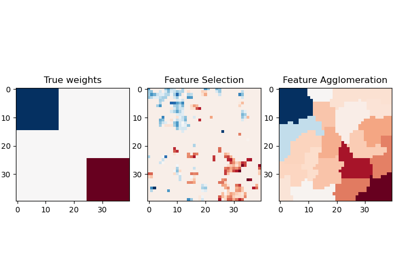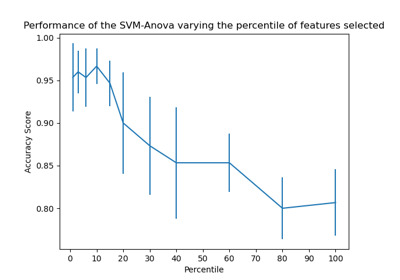# sklearn.feature_selection.SelectPercentile¶

class sklearn.feature_selection.SelectPercentile(score_func=<function f_classif>, *, percentile=10)[source]

Select features according to a percentile of the highest scores.

Read more in the User Guide.

Parameters
score_funccallable, default=f_classif

Function taking two arrays X and y, and returning a pair of arrays (scores, pvalues) or a single array with scores. Default is f_classif (see below “See also”). The default function only works with classification tasks.

New in version 0.18.

percentileint, default=10

Percent of features to keep.

Attributes
scores_array-like of shape (n_features,)

Scores of features.

pvalues_array-like of shape (n_features,)

p-values of feature scores, None if score_func returned only scores.

f_classif

ANOVA F-value between label/feature for classification tasks.

mutual_info_classif

Mutual information for a discrete target.

chi2

Chi-squared stats of non-negative features for classification tasks.

f_regression

F-value between label/feature for regression tasks.

mutual_info_regression

Mutual information for a continuous target.

SelectKBest

Select features based on the k highest scores.

SelectFpr

Select features based on a false positive rate test.

SelectFdr

Select features based on an estimated false discovery rate.

SelectFwe

Select features based on family-wise error rate.

GenericUnivariateSelect

Univariate feature selector with configurable mode.

Notes

Ties between features with equal scores will be broken in an unspecified way.

Examples

>>> from sklearn.datasets import load_digits
>>> from sklearn.feature_selection import SelectPercentile, chi2
>>> X.shape
(1797, 64)
>>> X_new = SelectPercentile(chi2, percentile=10).fit_transform(X, y)
>>> X_new.shape
(1797, 7)


Methods

 fit(X, y) Run score function on (X, y) and get the appropriate features. fit_transform(X[, y]) Fit to data, then transform it. get_params([deep]) Get parameters for this estimator. get_support([indices]) Get a mask, or integer index, of the features selected Reverse the transformation operation set_params(**params) Set the parameters of this estimator. Reduce X to the selected features.
fit(X, y)[source]

Run score function on (X, y) and get the appropriate features.

Parameters
Xarray-like of shape (n_samples, n_features)

The training input samples.

yarray-like of shape (n_samples,)

The target values (class labels in classification, real numbers in regression).

Returns
selfobject
fit_transform(X, y=None, **fit_params)[source]

Fit to data, then transform it.

Fits transformer to X and y with optional parameters fit_params and returns a transformed version of X.

Parameters
X{array-like, sparse matrix, dataframe} of shape (n_samples, n_features)

Input samples.

yndarray of shape (n_samples,), default=None

Target values (None for unsupervised transformations).

**fit_paramsdict

Returns
X_newndarray array of shape (n_samples, n_features_new)

Transformed array.

get_params(deep=True)[source]

Get parameters for this estimator.

Parameters
deepbool, default=True

If True, will return the parameters for this estimator and contained subobjects that are estimators.

Returns
paramsmapping of string to any

Parameter names mapped to their values.

get_support(indices=False)[source]

Get a mask, or integer index, of the features selected

Parameters
indicesbool, default=False

If True, the return value will be an array of integers, rather than a boolean mask.

Returns
supportarray

An index that selects the retained features from a feature vector. If indices is False, this is a boolean array of shape [# input features], in which an element is True iff its corresponding feature is selected for retention. If indices is True, this is an integer array of shape [# output features] whose values are indices into the input feature vector.

inverse_transform(X)[source]

Reverse the transformation operation

Parameters
Xarray of shape [n_samples, n_selected_features]

The input samples.

Returns
X_rarray of shape [n_samples, n_original_features]

X with columns of zeros inserted where features would have been removed by transform.

set_params(**params)[source]

Set the parameters of this estimator.

The method works on simple estimators as well as on nested objects (such as pipelines). The latter have parameters of the form <component>__<parameter> so that it’s possible to update each component of a nested object.

Parameters
**paramsdict

Estimator parameters.

Returns
selfobject

Estimator instance.

transform(X)[source]

Reduce X to the selected features.

Parameters
Xarray of shape [n_samples, n_features]

The input samples.

Returns
X_rarray of shape [n_samples, n_selected_features]

The input samples with only the selected features.

## Examples using sklearn.feature_selection.SelectPercentile¶